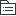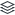# 第一章 物权总论

40
|
13

## 相关模板推荐

•## 物权总论 —— 作品大纲

•物权概念与特征
• 直接支配、对抗第三人
• 特征
• 直接支配物
• 依自己意思
• 义务人不特定，绝对权
• 使用价值是有形的
• 客体是物，有体物
• 直接享受物利益
• 利益
• 物的归属
• 物的利用
• 物的价值设立债务担保
• 排他性
• 排除权利干涉
• 同一物不许有内容不相容物权并存
•物权法概念与内容
• 调整人对物支配关系法律规范总和
• 内容
• 全面
• 利用
• 交换价值
• 占有
• 支配关系变动
• 产生，主体间
• 变更，客体、内容
• 消灭，事实发生
•效力
• 优先效力
• 矛盾权利并存，强权利排斥弱权利
• 物权相互间
• 能并存，先优于后
• 用、担可
• 用、用不可
• 担、担，可
• 限制物权优先所有权
• 物权对于债权
• 物优于债
• 同一标的物：买卖不破租赁
• 债权人依破产程序或强制执行程序行使债权，债务人财产物存在他人物权，物权优于一般债权
• 物上请求权
• 性质
• 请求权：妨害时，特定人为特定行为
• 物权的效用：恢复支配状态为目的
• 附属于物权
• 行使
• 直接提出物上请求权
• 请求返还原物
• 没有法律依据占有
• 原物灭失，请求赔偿
• 排除妨碍，消除危险
• 违法行为
•类型
• 物权法定主义
• 物权法体系中处于枢纽地位
• 规定种类、内容
• 要求
• 类型强制
• 内容固定
• 创设后果：不发生物权效力
• 种类
• 所有权
• 独占性支配
• 用益物权
• 使用、收益
• 担保物权
• 第三人特定财产设定物权
• 占有
• 控制、占领
• 民法上分类
• 自物、他物
• 动产、不动产
• 主物、从物：独立？
• 所有权、限制物权
• 有限期、无限期
• 时效针对债权而言
• 民法、特别法：特殊优先
• 本权、占有
•变动
• 概念
• 产生、变更、消灭
• 原则
• 公示
• 外部表现
• 动产，交付，运输工具除外
• 不动产，登记
• 公信
• 信赖，即使表现状态与真实状态不符，不影响变动效力
• 保护交易安全
• 善意取得，在法律规定条件下信赖公示却无权处分人取得物权
• 原因
• 取得
• 民事行为
• 买卖、互益、赠与
• 其他
• 善意取得
• 遗失物取得
• 征收
• 添附
• 没收
• 因合法生产建造
• 因法律文书
• 孳息：分红、果实、企业
• 消灭
• 民事
• 抛弃：单方
• 合同：约定物权存续、消灭
• 撤销权：法律、合同
• 其他
• 标的物灭失
• 法定期间届满
• 混同
• 同一物所有权归属一人，其他消灭
• 是为原则
• 模式
• 意思主义立法例：债权合同
• 形式主义
• 折中主义
• 公示方法
• 交付
• 转移占有
• 交付对抗主义
• 公示方法
• 交付要件主义
• 生效要件
• 通常指现实交付
• 简易交付
• 占有改定
• 指示交付：出让权利，代替交付
• 拟制交付：权利凭证
• 登记
• 效力
• 登记对抗主义
• 登记要件主义：变动要件，不登记不发生
• 不动产登记薄、登记机构、登记资料查询
• 产权证书，权利人
• 登记薄，登记机构
• 应当一致
• 不一致，除有证据证明登记薄确有错误，以登记薄为准
• 登记种类
• 更正登记
• 权利人、利害关系人
• 确有错误
• 直接申请
• 权利人书面同意或有证据证明登记错误，予以更正
• 异议登记
• 权利人不同意，利害关系人可申请
• 登记之日起十五日内不起诉，失效
• 造成不当，可申请赔偿
• 预告登记
• 所有权
• 集体土地
• 房屋所有权
• 用益物权
• 建设用地
• 宅基地使用
• 土地承包经营
• 海域
•保护
• 物权自我保护，即自力救济
• 请求权
• 民事诉讼程序，公力救济
• 特殊方法
• 请求确认物权
• 物上请求权：恢复对物的支配权
• 请求修理、重作、更换或恢复原状
• 请求赔偿损失：不能恢复原状